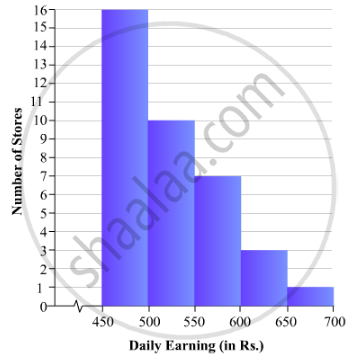# Draw a Histogram for the Daily Earnings of 30 Drug Stores in the Following Table: - Mathematics

Draw a histogram for the daily earnings of 30 drug stores in the following table:

 Daily earning(in Rs): 450-500 500-550 550-600 600-650 650-700 Number of Stores: 16 10 7 3 1

#### Solution

To represent the given data by a histogram, we first draw horizontal and vertical axes. Let us consider that the horizontal and vertical axes represent the class-intervals and the frequencies of the class-intervals respectively.

The given data is a continuous grouped frequency distribution with equal class-intervals. Construct rectangles with class-intervals as bases and respective frequencies as heights. The scale for horizontal axis may not be same as the scale for vertical axis. Let us take one vertical division is equal to 1 unit.

The heights of the different rectangles are as follows:

1. The height of the rectangle corresponding to the class-interval 450-500 is 16 big divisions.

2. The height of the rectangle corresponding to the class-interval 500-550 is 10 big divisions.

3. The height of the rectangle corresponding to the class-interval 550-600 is 7 big divisions.

4. The height of the rectangle corresponding to the class-interval 600-650 is 3 big divisions.

5. The height of the rectangle corresponding to the class-interval 650-700is 1 big divisions.

The histogram of the given data is as follows:Concept: Graphical Representation of Data
Is there an error in this question or solution?

#### APPEARS IN

RD Sharma Mathematics for Class 9
Chapter 23 Graphical Representation of Statistical Data
Exercise 23.3 | Q 7 | Page 42

Share